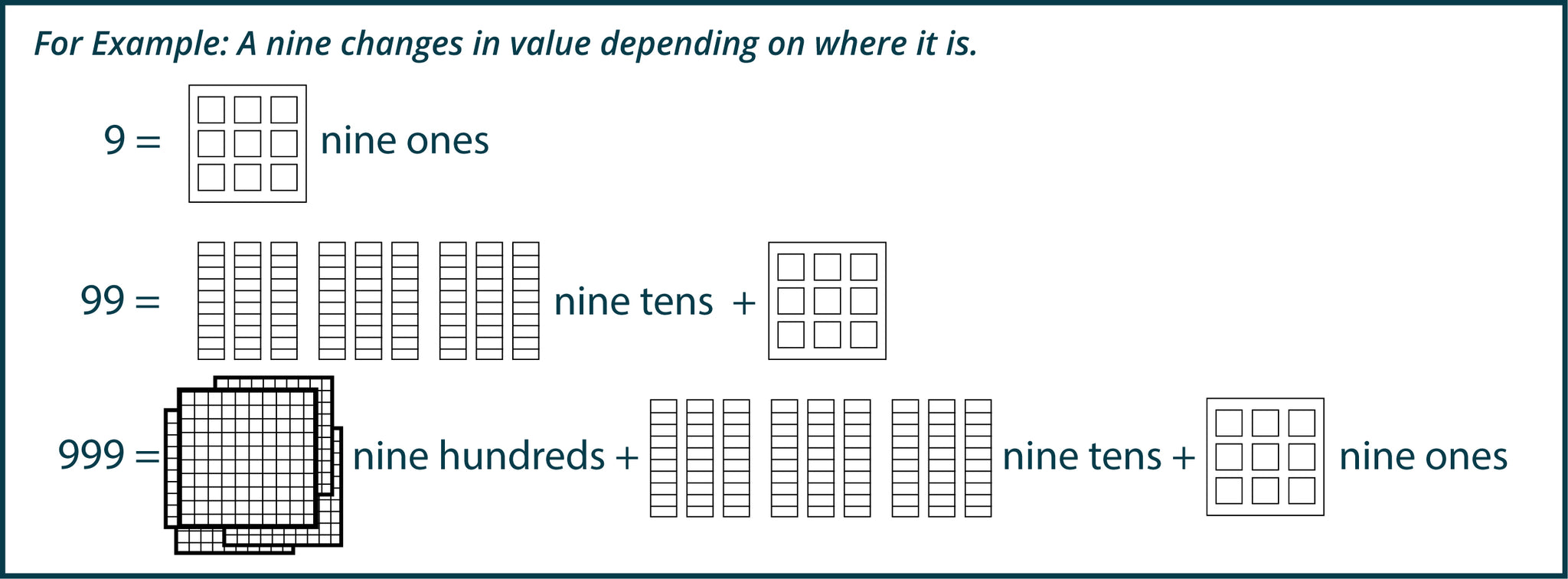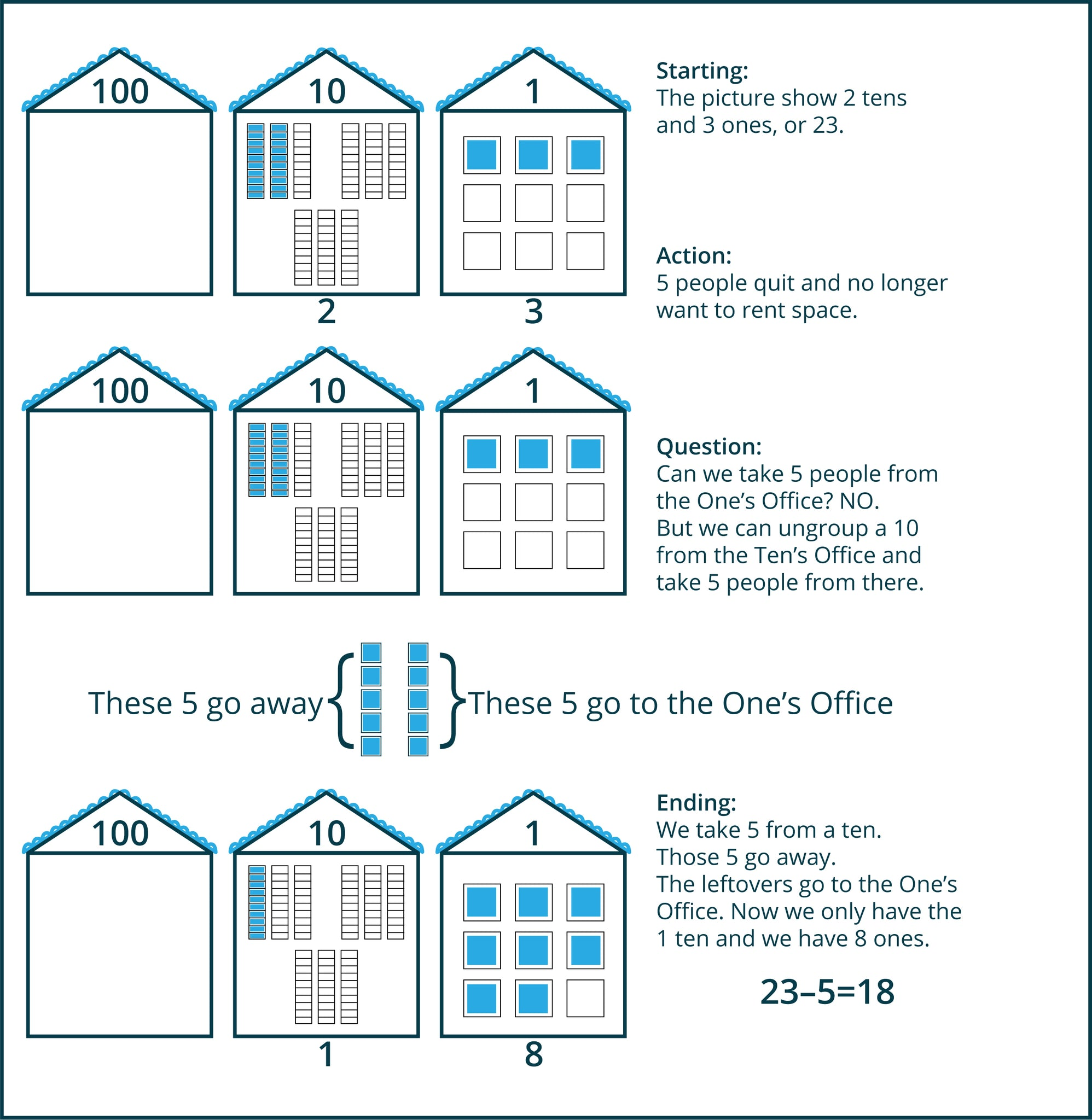How To Teach Right-Brained Addition & Subtraction Vol. 2

# How To Teach Right-Brained Addition & Subtraction Vol. 2

This User Guide is designed to give you a birds-eye view of the purpose of this book, outline the primary skills the students will gain, and provide a synopsis of those skills and how they differ from traditional approaches to adding and subtracting multi-digit numbers.

Many students who struggle with adding and subtracting numbers larger than one digit have trouble because they are not familiar with their math facts, they get lost when trying to follow the steps to solve a problem, or they are unable to remember those steps to begin with. Very often, also, students are so focused on remembering the steps to solve the problems that they don’t focus on, or maybe didn’t ever understand, what is actually happening in each problem.

Another barrier to success for these students is that it is fun for almost no one to learn, remember, and use steps. What IS fun and engaging is solving a problem when you understand the problem and feel competent to solve it.

The method this book lays out solves all these problems by simplifying the procedure and by avoiding the need to add numbers whose sum is greater than 10. The book also supplies ample hands-on and tactile/visual practice that shows the students what is happening so they can understand the point of the work.

These differences in approach are transformative for students who have struggled with math.

## What follows is a concise outline of the book

Hints for success

• Show the action (what is happening) in the problem we are solving. This will help children remember what to do to solve a similar problem in the future. This book does this for you.
• Allow children to practice the action and then settle into the method of solving the problem that works best for them. There is ample opportunity for hands-on practice in the book.

#### 3 concepts to understand & teach

• What does place value mean?
• What does it mean to make a ten?
• What does it mean to take from ten?

1. WHAT DOES PLACE VALUE MEAN?

What follows are the basic principles of place value:

A. When adding numbers with sums larger than 10, we will write the answers using digits 1-9 placed side by side. For example: 27, 326, 7632, 99081, 453678, and so forth.
B. Where these digits are placed in the lineup will show their value.C. Zeros help put numbers in the right place to show the correct value:
9 has no zero and its value is 9 ones.
90 has one zero that pushes the 9 left one place, and its value is 9 tens. (10s)
900 has two zeros that push the 9 left two places, and its value is 9 hundreds. (100s)
9000 has three zeros that push the 9 left three places, and its value is 9 thousands. (1000s)

D. Each digit in every multi-digit number has a place (“Office”):2. WHAT DOES IT MEAN TO MAKE A TEN?
What follows are the basic principles of making a ten:
A. When we add multi-digit numbers, our computation is based on making tens.
B. In any column of the problem, once we see that the numbers we are adding will be larger than 9, we will make a ten and move it left one place.
C. Making tens will only involve adding these combinations of numbers: 1+9, 2+8, 3+7, and 4+6.
D. Having a story will help cement what is going on and why we make tens.
Here is the story of making a ten:

“In this town there are different offices for different numbers of people. There is a Ones Office, a Tens Office, a Hundreds Office, and so forth.

In the Ones Office, you will find 9 desks that people rent. Once the 9 desks are full, if more people come in wanting to rent a desk, 10 of the people make a group and move into the Tens Office next door, occupying a long table together.

The Tens Office has 9 long tables that each seat 10 people. When those 9 tables are full, if another group comes in the door from the Ones Office, all ten groups bundle together to make a crowd of 100 people and together they move left into the Hundreds Office which has 9 rooms each containing 100 people.”*HINT: When making a ten, always start with the largest number and take what you need to make a ten from the smaller number. In this way, you will only ever need to add 6+4, 7+3, 8+2, or 9+1. You will also only ever be taking a 1, 2, 3, or 4 from another 1 digit number, which will go a long way toward making multi-digit addition & subtraction super kid friendly.

3. WHAT DOES IT MEAN TO TAKE FROM TEN?

What follows are the basic principles of taking from ten:

A. Let’s begin with the same set up we had when making tens. When we subtract, sometimes we are supposed to subtract a number from a number smaller than itself.
B. When this happens, we take a ten from the column to the left and unbundle it.
C. That leaves one fewer ten in the Tens Office.
D. We subtract the number from the ten we unbundled and that number goes away.
E. The leftovers are added to the Ones Office.To sum up: Using this approach children will only need to subtract 1-digit numbers from 10 and will only need to add 1-digit numbers that equal a number smaller than 10.

Right-Brained Addition & Subtraction, Vol. 2 details step-by-step exactly what to do, how to teach these concepts, and contains all the hands-on activities and student worksheets you will need.